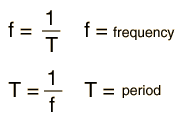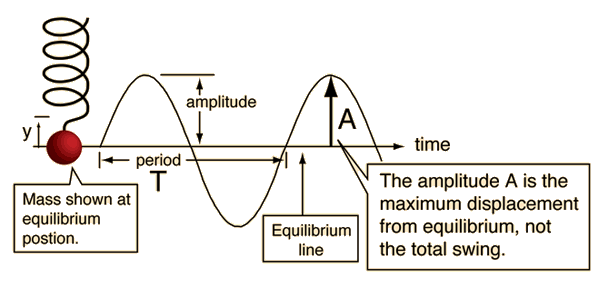# Periodic Motion

Periodic motion of some source object is necessary to produce a sustained musical sound (i.e., one with definite pitch and quality). For example, to produce a standard musical A (440 Hz), the source object must sustain periodic motion at 440 vibrations per second with a tolerance of less than 1 Hz -- the normal human ear can detect the difference between 440 Hz and 441 Hz. The conditions necessary for periodic motion are

1. elasticity - the capacity to return precisely to the original configuration after being distorted.
• a. a definite equilibrium configuration
• b. a restoring force to bring the system back to equilibrium
2. A source of energy.

Fortunately, it is not hard to find vibrators which meet these conditions, hence the richness in variety of musical sound sources.

### Terms for describing periodic motion.

A mass on a spring is an example of periodic motion with a single frequency called simple harmonic motion.
Index

Periodic motion concepts

Elasticity and musical sources

 HyperPhysics***** Sound R Nave
Go Back

# Description of Periodic Motion

Motion which repeats itself precisely can be described with the following terms:

• Period: the time required to complete a full cycle, T in seconds/cycle

• Frequency: the number of cycles per second, f in 1/seconds or Hertz (Hz)

• Amplitude: the maximum displacement from equilibrium A

and if the periodic motion is in the form of a traveling wave, one needs also

 Traveling wave relationship Traveling wave parameters
 Example: vary the parameters for a sine wave
Index

Periodic motion concepts

 HyperPhysics***** Sound R Nave
Go Back

# Period, Frequency and Amplitude

 In a plot of periodic motion as a function of time, the period can be seen as the repeat time for the motion. The frequency is the reciprocal of the period.If the period T = s T = ms T = x 10^ s then the frequency f = Hz f = kHz f = MHz f = x 10^ HzSimple harmonic motion Equilibrium condition
Index

Periodic motion concepts

 HyperPhysics***** Sound R Nave
Go Back